American Journal of Theoretical and Applied Statistics
Volume 6, Issue 1, January 2017, Pages: 32-37

A Model for Age-Specific Fertility Rate Pattern of India Using Skew-Logistic Distribution Function

Ruchi Mishra, Kaushalendra Kumar Singh, Anjali Singh

Department of Statistics, Institute of Science, Banaras Hindu University, Varanasi, India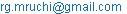(R. Mishra)

Ruchi Mishra, Kaushalendra Kumar Singh, Anjali Singh. A Model for Age-Specific Fertility Rate Pattern of India Using Skew-Logistic Distribution Function. American Journal of Theoretical and Applied Statistics. Vol. 6, No. 1, 2017, pp. 32-37. doi: 10.11648/j.ajtas.20170601.14

Received: December 26, 2016; Accepted: January 6, 2017; Published: February 3, 2017

Abstract: Fertility governs central and positive role in the study of human population dynamics. The age-specific fertility pattern has a distinct shape for all human population, to describe which, a number of parametric models have been proposed. The purpose of this study is to develop a mathematical model for fitting age-specific fertility rate pattern of various states of India. Skew-logistic probability density function is used for building the model. The real data, to which this model has been fitted, is obtained from National Family Health Survey- III (2005-2006). The used model is very flexible in nature and hence is useful for modeling diverse fertility patterns which are observed across different states of India. The parameters of the model have been estimated through the method of non-linear least square. By fitting the model it is observed that the proposed model fits well on the fertility pattern for almost each state of the country.

Keywords: Age-Specific Fertility Rate, Parametric Model, Skew-Logistic Probability Density Function, Non-Linear Least Square

Contents

1. Introduction

Fertility is one of the three main demographic features for any population (the others being mortality and migration). The growth of a human population depends solely on human fertility. Fertility represents the actual level of reproduction of a population based on the number of live births to a woman. Fertility levels are the determinant of the age structure of population which in turn governs the socio-economic and demographic characteristics of the population. Fertility, being a very complex phenomenon, affected by various social, biological, psychological, environmental and political factors, has always been a concern for demographers. Some of them were interested in finding the possible factors which govern fertility pattern such as age at marriage, gender preference, education, nutritional status of women, contraceptive use, current family size, desired family size, occupation, religion etc. and some were interested in finding estimates of direct (Crude Birth Rate, Age Specific Fertility Rate, General Fertility Rate, Total Fertility Rate, Gross Reproduction Rate, Net Reproduction Rate) and indirect (age-sex composition, Child-women ratio, female mean age at marriage) measures of fertility. The other way of measuring fertility is using mathematical functions (models) to assess the fertility pattern of any population by which one can reflect the true picture of it for any population. Various types of mathematical modeling such as parametric, non-parametric and polynomial models have been used in previous studies. Modeling is useful in analyzing fertility pattern as well as it also provides population projections, which might be helpful in framing government policies.

2. Modeling Age-Specific Fertility Rate Pattern

Generally, the typical fertility curve is somewhat bell-shaped, having its peak at around age 25 for females in reproductive age period. Fertility level starts slowly at around age 15, which is the beginning of the reproductive age, reaches its peak for the women of age group 25-30 years, and after that, starts to decline and has very low value for females after age 35 and usually ends at age 49, which is the end point of reproductive age span.

The general form of a fertility curve is given as follows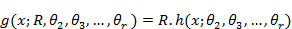(1)

where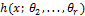is the probability density function (pdf) on real line having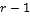parameters,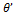s are the parameters of model, R,which is the rth parameter, is the total fertility rate (TFR). Using different functions in place oflike Beta and Gamma , Coale and Trussell [2,3], Inverse Gaussian, Hadwiger distribution [4, 5, 6] pdfs, different fertility models have been proposed by these authors. Some of these models, which represent the unimodal fertility pattern, show very good fitting to single year fertility rates . In addition to above functions, the Pearson Type I curve [7, 8] and Type III curves , the Brass procedures [10, 11], the Gompertz curve  and polynomial models  have also been applied on real data. Islam (2009)  has suggested a third degree polynomial model to fit the fertility pattern of Bangladesh which can be given as: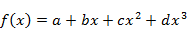(2)

Brijesh P. Singh et.al.  suggested a polynomial model with the inverse of x, to fit the fertility pattern of Uttar Pradesh (India) which can be mathematically represented as: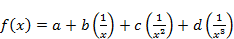(3)

Kaushalendra et. al.  compared different mathematical fertility models for India as a whole and some of its selected states. In recent years the fertility pattern of some developed countries has deviated from classical bell shape, it showed a little hump in the left part of fertility curve which may correspond to teenage fertility i.e. high fertility for early ages . Some authors have tried to give mathematical models which can fit the bimodal fertility curve of these countries which are having two modes, one in early age group and the other in age-group 25-30 years. Assuming the population as the mixture of two population exhibiting different fertility rates, Chandola et al.  has given the mixture of two Hadwiger function which has six parameters. The Hadwiger function is expressed as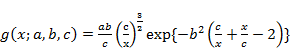(4)

provides a good fit to fertility pattern of modern population. It has three parameters. The Hadwiger mixture model is given as: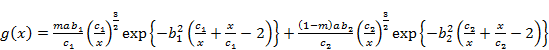(5)

Kohler  added an additional parameter to this model. Later, Peristera and Kostaki  suggested a mixture of normal distribution having different variance parameter before and after mean age which is represented as follows: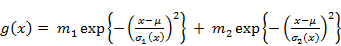(6)

Schmertmann  proposed piecewise quadratic spline function which shows very good fit to wide forms of fertility pattern but has 13 parameters. Azzalini  introduced a new type of skew-normal curve which includes the normal one. The model proposed by Azzalini has an extra parameter, setting which to 0 the curve reduces to normal density function. A Skew-Normal density is the generalization of Normal one with a skewness parameter. In general, the skew-normal density can be given as follows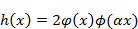(7)

where φ(x) is the pdf and Φ(x) is the cumulative distribution function (CDF) of standard normal distribution and α being the skewness parameter which is a real number. If α is taken as 0 then the above equation reduces to the density of standard normal distribution, thus the latter one is the particular case of skew-normal distribution.

The above model in (7) is unimodal curve . Mazzuca and Scarpa  used the generalized skew-normal, which is termed as Flexible Generalized Skew-Normal (FGSN) distribution to fit bimodal fertility schedule. The model is given as: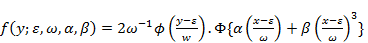(8)

The above model can have at most two modes, and as the degree of odd function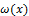increases, the number of modes allowed in the pdf increases. The skew-normal density is the key representative of skew-symmetric family. For more insight into the properties of this family, the readers may refer to Genton  and a review paper of Azzalini .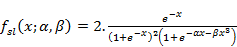(9)

The model (9) was used by Asili et. al.  for fitting age-specific fertility rates of Ireland and Greece. The fertility curve fitted by them has bimodal shape. They observed that this model fits better than skew-normal distribution used by Mazzucco and Scarpa .

The beautifulness of skew-symmetric density based fertility model lies in the fact that it can fit unimodal, bimodal as well as multi-modal fertility patterns effectively. The skewness parameter can change the symmetric curve into asymmetric one if it is required. Initially, it has three parameters, the number of skewness parameters can be increased accordingly to make the curve suitable for multi-modal fertility schedules. Thus, the skew-symmetrical models are flexible enough to fit a wide variety of fertility schedule. The previously proposed models are quite reliable but include some complexity in estimating the parameter(s) of the model. Here, in this study, the Skew-logistic probability distribution is used to study the age-specific fertility pattern of India.

3. Skew-Logistic Distribution Based Fertility Model

Azzalini  proposed a formula for skewing the symmetric distribution which is given as: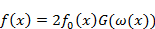(10)

Where,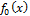is any symmetric probability density function,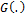is the cumulative distribution function of symmetric density and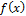is the density function for any odd function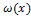.

The cumulative distribution function and probability density function of skew logistic distribution are as follows: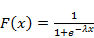(11)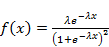(12)

Putting λ=1,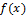in place of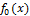and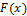in place of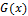and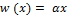in the equation (10) we get the skew-logistic distribution function as follows: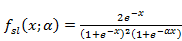(13)

where α is the skewness parameter and x R.

If we transform x into, in which µ is the location parameter and σ is the scale parameter, from (12) we get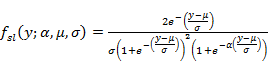(14)

Function in (14) is used to model the fertility pattern of India as it is observed that the Indian fertility curve has only one mode for almost all states (as shown in Figure 1) so the model (14) would be appropriate for Indian fertility schedule.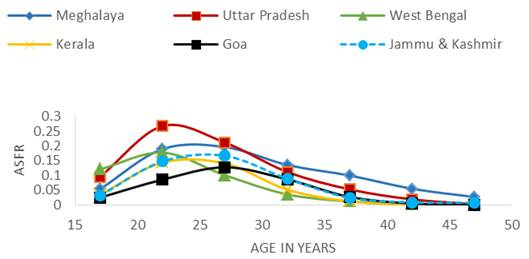Figure 1. Graph showing observed Fertility Pattern for females of Some States.

For estimating the parameters of fitted model the method of non-linear least square has been used. The estimated parameters are obtained by minimizing the residuals sum of squares which is mathematically represented by the following equation: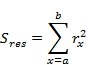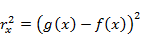for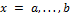where a and b are the lower and upper age limits of reproductive age span respectively, g(x) is the fertility rate at age x obtained by the proposed model and f(x) is the real or observed fertility rate at age x. From Figure 1 it is clear that Indian states have very diverse fertility pattern. States like Uttar Pradesh has high age-specific fertility rates for women in all reproductive age-groups while on the other hand states like Goa has low age-specific fertility rates for women belonging to each age-group in reproductive age span. Some states like Goa, Jammu & Kashmir, Meghalaya, Nagaland and Manipur have highest value of ASFR for females in age group 25-30 years while others have observed highest ASFR in age group 20-24 years. For the analysis as well as comparison purpose states are divided into two broad categories based on their value of TFR being high or low as compared to TFR for India which is 2.66 (NFHS-III). Further, some states have almost equal ASFR in the age groups 20-24 and 25-29 years. The fertility patterns exhibited in these states have a flat peak. These states are again grouped together in both categories. Table 1 shows the classification in four categories formed according to the fertility pattern of the state.

Table 1. Classification of Indian States as to their fertility level.

 States which have TFR>2.66 States which have TFR<2.66 Group 1* Group 2** Group 1* Group 2** Arunachal Pradesh, Meghalaya, Mizoram, Nagaland, Manipur Bihar, Chhattisgarh, Jharkhand, Madhya Pradesh, Rajasthan, Uttar Pradesh, Haryana Delhi, Goa, Jammu & Kashmir, Kerala Andhra Pradesh, Assam, Gujarat, Himachal Pradesh, Karnataka, Maharashtra, Orissa, Punjab, Sikkim, Tamil Nadu, Tripura, Uttarakhand, West Bengal

* For this group the fertility level is almost equal for females belonging to age-group 20-24 and 25-29years

** For this group the fertility level is different in age groups 20-24 and 25-29 years

By this classification, one may provide more clarity to the considered problem. Though it is observed that the model fits the fertility pattern for almost all states of India, in this study, one state from each group has been considered for analysis purpose. The selected states are Meghalaya, Uttar Pradesh, Kerala and West Bengal. The whole calculation and analysis are done through SOLVER in Microsoft Excel software, for graphics Microsoft Excel is used.

4. Result and Discussion

The fertility model considered in this paper is fitted to the real fertility data for various states of India.

The data has been obtained from National Family Health Survey- III (NFHS-III) . This is the latest report available on NFHS and hence appropriate for estimating the current fertility pattern. The age-specific fertility rate for reproductive age group i.e. 15-49 years has been taken for study purpose. This survey provides data and estimates for fertility, mortality, family planning practices, maternal and child health, reproductive health, HIV/AIDS and awareness, nutritional status, utilization and quality of health and family planning services across 29 states/union territories and also India as a whole. India is a diverse country in respect of fertility level. Some states have high fertility level and they are Bihar, Jharkhand, Haryana, Madhya Pradesh, Rajasthan and Uttar Pradesh (having TFR > 2.66). Goa, Kerala, Manipur, Sikkim and Tripura are the states having low fertility levels (having TFR < 2.66). Some states like West Bengal have high level of fertility for the females in of early age group i.e. 15-19 years age interval. This may be because of the prevailing custom of early age marriage in these states.

Table 2 shows the actual and fitted age-specific fertility rates for women in age group 15-49 years for the states Meghalaya, Uttar Pradesh, Kerala and West Bengal.

Table 2. Observed and Estimated values of ASFR for the Different States.

 Meghalaya Uttar Pradesh West Bengal Kerala Age-group(in Years) Mid-Value (x) Observed ASFR Estimated ASFR Observed ASFR Estimated ASFR Observed ASFR Estimated ASFR Observed ASFR Estimated ASFR 15-19 17 0.054 0.054 0.096 0.096 0.121 0.121 0.035 0.035 20-24 22 0.190 0.190 0.268 0.268 0.178 0.178 0.144 0.144 25-29 27 0.197 0.195 0.212 0.213 0.101 0.101 0.141 0.141 30-34 32 0.136 0.142 0.112 0.111 0.036 0.037 0.052 0.051 35-39 37 0.100 0.092 0.053 0.050 0.012 0.011 0.012 0.013 40-44 42 0.055 0.055 0.019 0.021 0.003 0.003 0.001 0.003 45-49 47 0.027 0.032 0.003 0.009 0.003 0.001 0.000 0.001 TFR 3.79 3.80 3.81 3.84 2.27 2.26 1.93 1.94

It is clear from the Table 2 that for all states in this study, the highest fertility level is observed for the females of age group 20-24 years and the lowest one is observed for the females of age group 45-49 years. After observing Table 1 and Figure 1, we see that the pattern of ASFR is unimodal for all states, it increases slowly from age 15 years, reaches its peak for the females of age group 20-24 years, and then again it starts to decline sharply and reaches to zero for the females after age 50 years. These states have a different level of fertility in different age-groups and have different TFR. Among these states, Uttar Pradesh has the highest level of fertility and Kerala has the lowest level of prevailing fertility. It is also observed that though Meghalaya and Uttar Pradesh have almost equal level of TFR, their ASFR for different age-groups are totally different. Uttar Pradesh has a high level of fertility in early age-groups whereas Meghalaya has a high level of fertility in late age-groups. Figures 2, 3, 4 and 5 show the fitting of the model to the real age-specific fertility rates of Meghalaya, Uttar Pradesh, Kerala and West Bengal respectively. Among these states, West Bengal has the highest level of early-age specific fertility and Kerala has the lowest level of early-age specific fertility. From the figures, it is clear that the model has a very good fit for the fertility pattern of women of all considered states.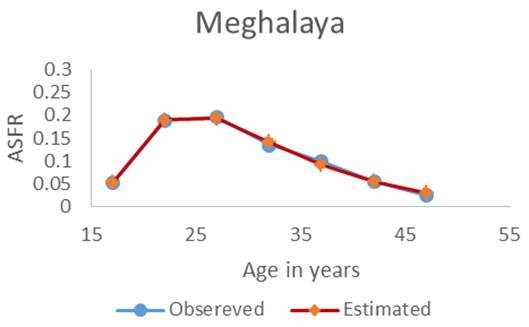Figure 2. The Plot of Observed and Estimated ASFR of Meghalaya.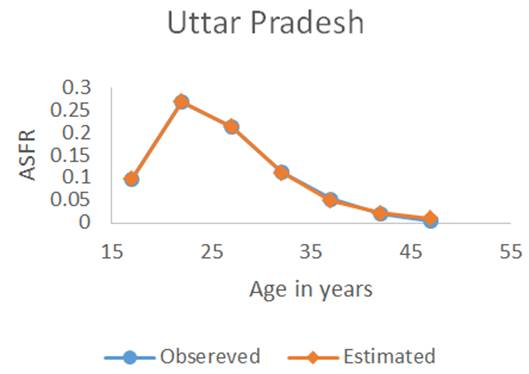Figure 3. The Plot of Observed and Estimated ASFR of Uttar Pradesh.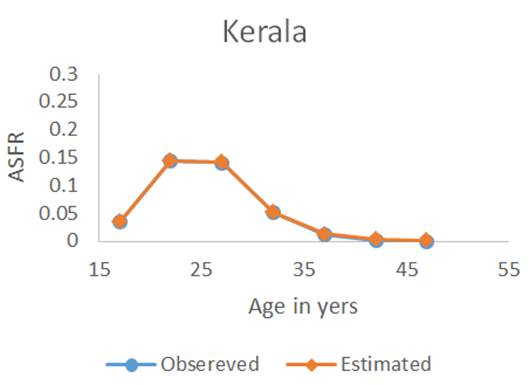Figure 4. The Plot of Observed and Estimated ASFR of Kerala.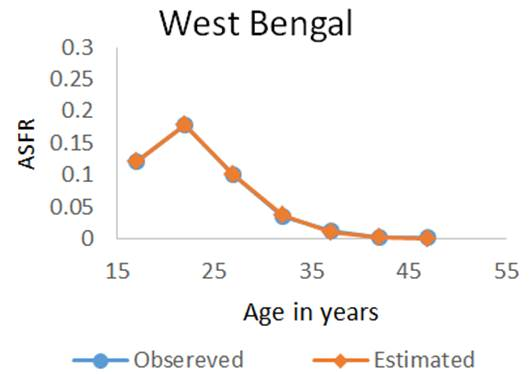Figure 5. The  Plot of Observed and Estimated ASFR of West Bengal.

India is a very diverse country concerning fertility pattern. In some states fertility is still very high and in some states, it has declined considerably. After analyzing the model for each state in the study, we see that the model fits for each state in all the categories despite the high or low level of prevailing fertility pattern.

From the Table 2, it is clearly evident that the actual and estimated fertility are very close to each other, which shows the good fitting of the model for Indian fertility schedule. The goodness of fitting for any model can be checked by various techniques and tools. Some of them are, comparing the current model with existing models which have been proven for their good fitting. Here, the method of non-linear least square is used for model fitting, so the value of the sum of squares due to residuals is taken as an indicator for model fitting. The less is the sum of squares due to residuals; the better is fitting of the model to given data. Table 3 shows the error sum of squares from the least square method for states in all four categories.

Table 3. Error Sum of Squares for the Different States.

 Sr. No. States Error sum of square 1. Meghalaya 1.34E-04 2. Uttar Pradesh 4.72E-05 3. Kerala 5.39E-06 4. West Bengal 4.83E-06

From the Table 3, we see that the sum of squares due to residuals is less than of order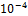for all states. Thus, indicating a good fit to the fertility pattern of these states.

The quality of demographic models not only depends on how good they fit the data but also on the demographic explanation of the parameters used in the model. The parameters of the Skew-logistic model are not of straightway explanation. The location parameter is not the mean of the distribution; it is a linear function of mean age at first birth. Similarly, the scale parameter is not the variance of the distribution; it can be explained as a function of the standard deviation of age at first birth of females in the study. Nothing can be explicitly said about α, the skewness parameter. Table 4 shows the estimated parameters of the model for each state.

Table 4. Estimated Parameters of the Model for the Different States.

 States Location Parameter (µ) Scale Parameter (σ) Skewness Parameter (α) TFR Kerala 22.31 3.26 0.79 1.95 Meghalaya 19.37 8.23 4.29 4.02 Uttar Pradesh 18.94 5.53 2.69 3.91 West Bengal 18.16 4.09 1.15 2.40

From Table 4, it can be observed that for each state, the skewness parameter α comes out to be positive which is obvious because fertility curve is positively skewed. It is also observed that the value of α lies within 0 to 5.

5. Conclusion

It is clearly observed from the tables and figures that the estimated values from the proposed model fits the data of observed fertility pattern for almost all Indian states considered. The observed age-specific fertility rates obtained by the NFHS-III data are very close to the values estimated by the proposed model. The advantage of this model is that it is very flexible and shows a very good fitting to different fertility patterns. India is very diverse country, the demographic profile of its states varies from state to state, but this model fits the age-specific fertility rate patterns of all states effectively. The previously proposed models have some complexity in terms of estimating the parameters of the model and their interpretation. As the Hadwiger mixture model incorporates with estimating six parameters. The model used in this study deals with only four parameters thus, it is better from the previously proposed models in this sense. Moreover, the model considered here is more flexible than other proposed models. The advantage of using skew-logistic model is that it can fit a variety of fertility patterns observed in the human population. It is equally appropriate for unimodal as well as multi-modal fertility schedule.

References

1. J. M. Hoem, D. Madsen, J. L. Nielsen, E. - M. Ohlsen, H. O. Hansen, and B. Ren-nermalm, "Experiments in modelling recent Danish fertility curves," Demography, vol. 18, no. 2, pp. 231–244, 1981.
2. A. J. Coale and T. J. Trussel. "Model fertility schedules: variations in the age structure of childbearing in human populations", Population Index, vol. 40, no. 2, pp. 185-258, 1974.
3. A. J. Coale and T. J. Trussel. "Technical note: finding the two parameters that specify a model schedule of marital fertility", Population Index, vol. 44, no. 2, pp. 203-214, 1978.
4. H. Hadwiger. "Eine Analytische Reprodutions-Funktion fur Biologische Gesamtheiten", Skandinavisk Aktuareitidskrift, vol. 23, pp. 101-113, 1940.
5. E. Gilje. "Fitting Curves to Age-Specific Fertility Rates: Some Examples", Statistical Review of the Swedish National Central Bureau of Statistics, vol. III, no. 7, pp. 118-134, 1969.
6. L. Yntema. "On Hadwiger’s fertility function", Statistical Review of the Swedish National Central Bureau of Statistics, vol.III, no. 7, pp. 113-117, 1969.
7. S. Mitra. "The Pattern of Age-Specific Fertility Rates", Demography, vol. 4, pp. 894-906, 1967.
8. A. Romanuik. "A Three Parameter Model for Birth Projections", Population Studies, vol. 27, no. 3, pp. 467-478, 1973.
9. M. N. Islam and S. A. Mallick. "On the Use of a Truncated Personian Type III Curve in Fertility Estimation", Dhaka Universities Studies Part B Science, vol. 35, no. 1, pp. 23-32, 1987.
10. W. Brass. "Perspectives in Population Prediction: Illustrated by the Statistics of England and Wales (with discussion)", Journal of the Royal Statistical Society, vol. 137, pp. 532-583, 1974.
11. W. Brass. "Population Projections for Planning and Policy", Papers of the East- West Population Institute, no. 5, 1978.
12. E. M. Murphy and D. M. Nagnur. "A Gompertz fit that fits: Applications to Canadian Fertility Pattern", Demography, vol. 9, pp. 35-50, 1972.
13. W. Brass. "The Graduation of Fertility Distributions by Polynomial Functions", Population Studies, vol. 14, pp. 148-162, 1960.
14. R. Islam. "Mathematical modeling of age specific marital fertility rates of Bangladesh", Research Journal of Mathematics and Statictics, vol. 1, pp. 19–22, 2009.
15. Brijesh P. Singh, Kushagra Gupta and K. K. Singh. "Analysis of fertility pattern through mathematical curves", American Journal of Theoretical and Applied Statistics, vol. 4, pp. 64-70, 2015.
16. Kaushalendra K. Singh, Anjali Singh and Anjali Pandey. "Modelling Fertility Curves In India: A Comparison of Four Mathematical Models", Janasamkhya Volume XXX & XXXII, pp. 13-29, 2012-2014.
17. P. Peristera and A. Kostaki, "Modeling fertility in modern populations," Demographic Research, vol. 16, pp. 141–194, 2007.
18. D. A. Coleman, T. Chandola and R. W. Hiorns. "Recent European fertility patterns: fitting curves to ’distorted’ distributions", Population Studies, vol. 53, no. 3, pp. 317–329, 1999.
19. Ortega Osona, J. A. and H. -P. Kohler, "A comment on "Recent European fertility patterns: Fitting curves to ‘distorted’ distributions" by T. Chandola, D. A. Coleman and R. W. Hiorns", Population Studies, vol. 54, pp. 347–349, 2000.
20. C. Schertmann. "A System of model fertility schedules with graphical intuitive parameters", Demographic Research, vol. 9, pp. 81-110, 2003.
21. A. Azzalini, "A class of distributions which includes the normal ones," Scandinavian Journal of Statistics, vol. 12, pp. 171–178,1985.
22. Y. Ma and M. G. Genton. "Flexible class of skew-symmetric distributions", Scandinavian Journal of Statistics, vol. 31, pp. 459-468, 2004.
23. S. Mazzuco and B. Scarpa. "Fitting age-specific fertility rates by a skew-symmetric probability density function", Working Paper Series Department of Statistical Science, University of Padua, 2011.
24. A. Azzalini, "Rejoinder," Scandinavian Journal of Statistics, vol. 32, no. 2, pp. 199–200, 2005.
25. National Family Heath Survey, Government of India, 2005-2006.
26. S. Asili, S. Rezaei and L. Najjar. "Using skew-logistic probability density function as a model for age-specific fertility rate pattern", BioMed Research International, vol. 2014, 2014, http://dx.doi.org/10.1155/2014/790294.

 Contents 1. 2. 3. 4. 5.
Article ToolsAbstractPDF(295K)